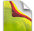### Feel The Power# PHP Expressions and Operators

In PHP there are two types of expression for us to look at. One is something called a "regular expression", which is used in the manipulation of text. We'll talk more about them later in the series. The other is more mathematical in nature, expressing operations to be performed on values and variables. Here's a simple example:

c = a + b

Assuming a and b are numbers (remember data types?  -- the result would be different if they were strings -- more about that later) this expression would add a and b and put the sum into c (remember again, that the equals sign here makes this an assignment instruction.)  In the expression a + b the plus sign is an arithmetic operator.  Here are some more operators:

Arithmetic Operators:

 + Addition Add two values - Subtraction Subtract the second value from the first * Multiplication Multiply two values / Division Divide the first value by the second % Modulus Divide the first value by the second and return only the remainder (for example, 7 % 5 yields 2)

Comparison Operators:

 = = Equal Checks for equal values = = = Identical Checks for equal values and data types ! = Not Equal Checks for values not equal ! = = Not Identical Checks for values not equal or not the same data type < Less than Checks for one value being less than the second > Greater than Checks for one value being greater than the second < = Less than or Equal to Checks for on value being less than or equal to the second > = Greater than or Equal to Checks for on value being greater than or equal to the second

Logical Operators:

 And Checks if two or more statements are true && Same as And Or Checks if at least one of two statements is true || Same as Or Xor Checks if only one of two statements is true ! Checks if a statement is not true

Increment and Decrement Operators:

 ++value Pre-Increment Adds 1 to the value before processing the expression which uses the value --value Pre-Decrement Subtracts 1 from the value before processing the expression which uses the value value++ Post-Increment Adds 1 to the value after processing the expression which uses the value value-- Post-Decrement Subtracts 1 from the value after processing the expression which uses the value

The Concatenate Operator

I get in trouble all the time because I ask how this can be considered an "operator", but trust e, the world thinks it is!  The concatenate "operator" is a period (full stop to the English English!)  It simply says "join this to this", so that

\$parta = "This is part one "
\$partb = "of a sentence!"

print \$parta.\$partb

prints out "This is part one of a sentence!"

"Huh?" I say!  "That's not a part of a sentence -- that's a whole sentence!!" (Please forgive my pedantic humor!)

Combined Operators:

There are some combination operators that you may see used (or may use!)  There are those who argue that these combination operators provide an advantage because they reduce typing and reduce the final size of the code.  In my humble opinion, however, they don't represent the way that most of us think of things, and are therefore somewhat confusing.  See what you think.

You remember from earlier examples that = is an assignment operator:

\$a = 5

assigns the value 5 to \$a.   This assignment can be combined with some other operators like this:

\$a += 5

If \$a already had a value of 5, then after this instruction it would be 10, as if the full instruction was:

\$a = \$a + 5

Valid combinations include:  +=  -=  *=  /=

Precedence and Associativity.

\$a = 7 - 4 + 1

If the minus happens before the plus, the result would be 4.  If the plus happens first, the result is 2.  Since predictability is essential, an order of precedence was established, along with something called associativity.  The order of precedence establishes a "rank" for operators, where those with the highest rank will be evaluated first.  When there are several operators of the same rank, they will be processed from left to right if they are left associative, and right to left if they are right associative.  Some operators, such as the comparison operators, have neither left nor right associativity.

The increment and decrement operators  ( ++ and -- ) are right associative, while the others I have discussed here are left associative.  The order of precedence, from highest to lowest (with those of equal precedence on the same line) is as follows:

++  --
*  /  %
+  -
|
&&
| |
and
xor
or

You can override the order of precedence but wrapping part of an expression in parentheses.  The innermost parentheses are processed first, so that:

(7 - 4) + 1 will give a result of 4
7 - (4 + 1) will give a result of 2

2 + 3 * 3 will give a result of 11
2 + (3 * 3) will also give 11
(2 + 3) * 3 will give a result of 15

1 + 3 + 3 * 2 will give a result of 10
1 + ((3 + 3) * 2) will give a result of 13
(1 + (3 + 3)) * 2 will give a result of 14

I like to use parentheses whenever I create a complex expression so that it is easier for me to see what's going on when I come back to some code that I originally wrote months or years ago.  Following this simple rule makes your code closer to self documenting and make you your own best friend!

#

#

#

### PHP Expressions Operators Definitions

TOP TUTORIALS
WHAT IS NEW IN KERIXA?
RECENT TUTORIALS
CATEGORIES